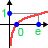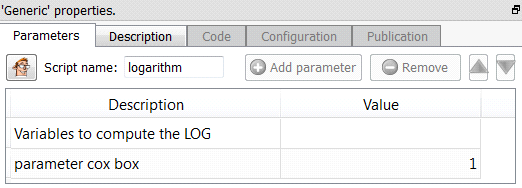﻿ 5. Detailed description of the Actions > 5.7. Data Mining > 5.7.6. Logarithm

# 5.7.6. Logarithm

Icon:Function: logarithm

Property window:Short description:

Compute the logarithm of many different Columns.

Long Description:

The logarithm of the selected columns is computed in the following way:

Out= (  (C+In)>0 ? log(C+In) :  -1  )

…where: “In” is the input number

“Out” is the result

“C” is the parameter cox box.

“x?y:z” is the classical ternary operator.

Transforming monetary values using a log function can sometime improve the accuracy of the continuous predictive models using these monetary values.